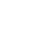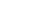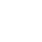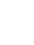# Total Debt Formula: How to Calculate Your Debt

## Understanding your total debt can go a long way in determining your net worth and planning for the future.Contributing Writer at Tally

June 20, 2022

There’s no shortage of formulas you can use to help determine your financial well-being. Some directly help to determine financial health, such as the net worth formula, but others are best used to supplement other formulas.

An example of the latter is the total debt formula. While it can help you get a 36,000-foot view of your overall debt and help you plan for the future, the total debt formula also plays a supporting role in determining other financial ratios.

So what is the total debt formula, and how does it support other financial formulas? We cover that and more below.

## What is the total debt formula, and why does it matter?

The total debt formula is a look into all the long-term and short-term debts you have. This formula is important because it gives you an overhead look at all your debts due within the year and those that stretch beyond a year. The results allow you to make debt-repayment plans and set a budget.

The total debt formula is also important because it plays a critical role in determining your total liabilities for use in your personal balance sheet.

As mentioned, within the total debt formula are short- and long-term debts. Let’s look at how each is defined and some examples.

### What is a short-term debt?

Short-term debt is anything you expect to pay in full within 12 months. Some examples of short-term liabilities or current liabilities include:

• Short-term loans

• Bank fees

• Credit cards

• Monthly subscriptions

• Utility bills

• Cell phone bill

• Current-year income taxes

• Accounts payable

### What is a long-term debt?

A long-term debt is any outstanding debt that will take longer than 12 months to pay off. Some examples of long-term liabilities include:

• Home mortgage

• Auto loan

• Long-term loans

• Back taxes

• Personal loans

• Lines of credit

## How do you calculate total debt?Calculating total debt is relatively simple. You collect all your long-term debts and add their balances together. You then collect all your short-term debts and add them together too. Finally, you add together the total long-term and short-term debts to get your total debt.

So, the total debt formula is: Long-term debts + short-term debts.

For example, let’s say you have the following liabilities (debts).

Short-term debts:

• Bank fees (\$15 per month): \$180

• Short-term loan: \$1,500

• Credit cards: \$5,000

• Electric bill (\$200 per month): \$2,400

• Water bill (\$100 per month): \$1,200

• Monthly streaming services (\$100 per month): \$1,200

Long-term debts:

• Auto loan: \$25,000

• Mortgage: \$150,000

• Personal loan: \$25,000

In this case, your short-term debts would equal \$11,480, and your long-term debts would be \$200,000. The total debt formula would be \$11,480 + \$200,000 = \$211,480. So your total debt or total liabilities would be \$211,480.

## How would you put your total debt formula to work?

There are a few ways to put your total debt formula to work and improve your personal finances. Here are a few common ways to use this information.

### Calculating net worthOne of the most common uses for the total debt formula is calculating your net worth. To determine your net worth after calculating the total debt formula, you must add up all your current assets, such as:

• Home value

• Vehicle values

• Savings account balances

• Checking account balances

• Investment account balances

• Retirement plan balances

• Accounts receivable amounts

• Cash equivalents on hand

• Cash on hand and working capital

You then subtract the result of your total debt formula from the total assets you calculated. This gives you your net worth.

For example, if you had a home worth \$300,000, a vehicle worth \$30,000, and account balances totaling \$50,000, you’d have \$380,000 in assets. Then, if you were to subtract the total amount of debt calculated above (\$211,480), you’d have a net worth of \$168,520 (\$380,000 - \$211,480 = \$168,520).

Net worth is important because it highlights the amount of money you would have access to if you sold all your assets and repaid all your debt obligations. Lenders often consider this a key metric when determining bank loan approval.### Calculating debt-to-equity ratio

When applying for credit, some lenders will also consider your debt-to-equity ratio (DTE ratio) as a measure of financial health and may reject someone with too high of a DTE ratio. The total debt formula will also play a role in determining your DTE ratio.

You can calculate your DTE ratio using the DTE ratio formula, which is total liabilities (total debt) divided by total equity. Using the same numbers we used to determine net worth, your total assets will be the total equity, so the DTE formula would be \$211,480 / \$380,000 = 0.556. Multiply the result by 100 and express it as a percentage, so your DTE ratio in this example would be 55.6%.

### Building your personal balance sheet

Another common use for the total debt formula is to build your personal balance sheet. Like a company’s balance sheet, a personal balance sheet is a three-step process, where you add up your assets, add up your liabilities, then calculate your net worth.

The total debt formula comes in handy in the second step: adding up your liabilities. You can use this formula to come up with the raw total of your liabilities. Your personal balance sheet then goes a little deeper into your financial obligations by including interest rates and monthly payments too, but you can obtain these while gathering your total debt balances from your financial statements.

Once you’ve laid out your personal balance sheet, you can use it in various ways. You can identify areas of financial stress and work toward alleviating them. You can get a broad overhead view of your finances and make changes to your financial plans based on what the balance sheet shows. Finally, you can use this sheet to determine your retirement plans, kids’ college funding, a debt-repayment plan and more.

## Visualize your financial obligations with the total debt formulaThe total debt formula, which adds up all your current and long-term liabilities or debts, gives you a bird’s-eye view of your financial obligations. This allows you to see where you may be overspending and where you can make adjustments to your financial strategy. Plus, it plays a role in determining your net worth and building your personal balance sheet — two important parts of maintaining your financial health.